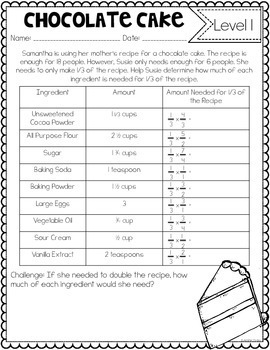# Fractions Tasks and Challenges {Differentiated Fraction Activities}5th - 6th
Subjects
Standards
Resource Type
Formats Included
• PDF
Pages
35+

### Description

Fractions made engaging (and delicious) with these chocolate-themed differentiated fraction activities!

*This engaging no-prep fractions resource contains 16 differentiated fraction tasks or challenges.

*Each task or challenge is presented in a word problem format. Many of the challenges contain multiple steps and/or tables to help organize information presented.

•Level 1: This level is the simpler level. This level may contain simpler numbers (Examples: like denominators, smaller factors, etc.), models, equation scaffolds, or thinking prompts. Some of these would work well for 4th grade students but most are better suited for struggling students in 5th and 6th grade.

•Level 2: This is an on-grade level task or challenge that is geared toward 5th grade standards.

These tasks all have a chocolate theme making them more engaging for students. Topics of the challenges include cookies, cake, donuts, and chocolate covered strawberries, to name a few!

These tasks could be used for RTI, assessment, centers, enrichment, etc!

Fraction Skills Included:

• Mixed Numbers, Improper Fractions, Simplifying, and Equivalent Fractions
• Comparing Fractions, Adding and Subtracting Fractions
• Comparing Fractions, Adding and Subtracting Fractions
• Adding and Subtracting Mixed Numbers
• Adding and Subtracting Mixed Numbers
• Multiplying Fractions by Whole Numbers
• Multiplying Fractions by Whole Numbers
• Multiplying Fractions, Multiplying Mixed Numbers
• Multiplying Fractions, Multiplying Fractions by Whole Numbers
• Multiplying Fractions
• Multiplying Mixed Numbers, Area with Fractional Sides
• Dividing Unit Fractions by Whole Numbers, Comparing Fractions
• Dividing Whole Numbers by Unit Fractions
• Adding Fractions, Dividing Whole Number by Unit Fractions
• Multiplication as Resizing

More Fraction Activities

Multiplying and Dividing Fractions Resources

Fraction Practice Pages

Fraction Spinner Math Centers

Adding and Subtracting Mixed Numbers Resources

Subtracting Mixed Numbers with Regrouping Resources

Fraction Math Mat Centers

Total Pages
35+
Included
Teaching Duration
2 months
Report this Resource to TpT
Reported resources will be reviewed by our team. Report this resource to let us know if this resource violates TpT’s content guidelines.

### Standards

to see state-specific standards (only available in the US).
Add and subtract fractions with unlike denominators (including mixed numbers) by replacing given fractions with equivalent fractions in such a way as to produce an equivalent sum or difference of fractions with like denominators. For example, 2/3 + 5/4 = 8/12 + 15/12 = 23/12. (In general, 𝘢/𝘣 + 𝘤/𝘥 = (𝘢𝘥 + 𝘣𝘤)/𝘣𝘥.)
Solve word problems involving addition and subtraction of fractions referring to the same whole, including cases of unlike denominators, e.g., by using visual fraction models or equations to represent the problem. Use benchmark fractions and number sense of fractions to estimate mentally and assess the reasonableness of answers. For example, recognize an incorrect result 2/5 + 1/2 = 3/7, by observing that 3/7 < 1/2.
Apply and extend previous understandings of multiplication to multiply a fraction or whole number by a fraction.
Interpret the product (𝘢/𝘣) × 𝘲 as a parts of a partition of 𝘲 into 𝘣 equal parts; equivalently, as the result of a sequence of operations 𝘢 × 𝘲 ÷ 𝘣. For example, use a visual fraction model to show (2/3) × 4 = 8/3, and create a story context for this equation. Do the same with (2/3) × (4/5) = 8/15. (In general, (𝘢/𝘣) × (𝘤/𝘥) = 𝘢𝘤/𝘣𝘥.)
Find the area of a rectangle with fractional side lengths by tiling it with unit squares of the appropriate unit fraction side lengths, and show that the area is the same as would be found by multiplying the side lengths. Multiply fractional side lengths to find areas of rectangles, and represent fraction products as rectangular areas.